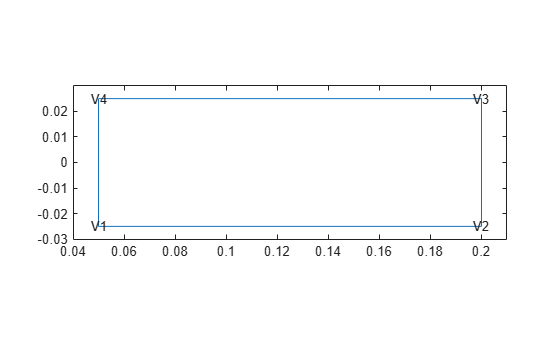# vertexBC

Boundary conditions on geometry vertex

Since R2023a

## Description

A `vertexBC` object specifies the type of boundary condition on a vertex of a geometry. An `femodel` object contains an array of `vertexBC` objects in its `VertexBC` property.

## Creation

### Syntax

``model.VertexBC(VertexID) = vertexBC(Name=Value)``

### Description

example

````model.VertexBC(VertexID) = vertexBC(Name=Value)` creates a `vertexBC` object and sets properties using one or more name-value arguments. This syntax assigns the specified structural boundary condition to the specified vertices of the geometry stored in the `femodel` object `model`. For example, `model.VertexBC([2,5]) = vertexBC(Constraint="fixed")` specifies that edges 2 and 5 are fixed boundaries.```

### Input Arguments

expand all

Vertex IDs, specified as a vector of positive integers. Find the vertex IDs using `pdegplot` with the `VertexLabels` value set to `"on"`.

Data Types: `double`

## Properties

expand all

Standard structural boundary constraint, specified as `"fixed"`.

Data Types: `char` | `string`

x-component of enforced displacement, specified as a real number or function handle. The function must return a row vector. Each element of this vector corresponds to the x-component value of the enforced displacement at the boundary coordinates provided by the solver. For a transient or frequency response analysis, `XDisplacement` also can be a function of time or frequency, respectively. For details, see Nonconstant Parameters of Finite Element Model.

For axisymmetric models, this property contains the radial component of the enforced displacement.

Data Types: `double` | `function_handle`

y-component of enforced displacement, specified as a real number or function handle. The function must return a row vector. Each element of this vector corresponds to the y-component value of the enforced displacement at the boundary coordinates provided by the solver. For a transient or frequency response analysis, `YDisplacement` also can be a function of time or frequency, respectively. For details, see Nonconstant Parameters of Finite Element Model.

For axisymmetric models, this property contains the axial component of the enforced displacement.

Data Types: `double` | `function_handle`

z-component of enforced displacement for a 3-D model, specified as a real number or function handle. The function must return a row vector. Each element of this vector corresponds to the z-component value of the enforced displacement at the boundary coordinates provided by the solver. For a transient or frequency response analysis, `ZDisplacement` also can be a function of time or frequency, respectively. For details, see Nonconstant Parameters of Finite Element Model.

Data Types: `double` | `function_handle`

## Examples

collapse all

Specify fixed boundary conditions for an `femodel` object representing an axisymmetric static structural problem.

Create a rectangular geometry that represents a spinning disk. The inner radius of the disk is 0.05, and the outer radius is 0.2. The thickness of the disk is 0.05.

`gm = decsg([3 4 0.05 0.2 0.2 0.05 -0.025 -0.025 0.025 0.025]');`

Plot the geometry with the vertex labels.

```pdegplot(gm,VertexLabels="on"); xlim([0.04 0.21]) ylim([-0.03 0.03])```Create an `femodel` object for solving a static axisymmetric structural problem, and assign the geometry to the model.

```model = femodel(AnalysisType="structuralStatic", ... Geometry=gm); model.PlanarType = "axisymmetric";```

Fix the axial displacement at vertices 1 and 4.

```model.VertexBC([1 4]) = vertexBC(Constraint="fixed"); model.VertexBC```
```ans = 1x4 vertexBC array Properties for analysis type: structuralStatic Index Constraint XDisplacement YDisplacement 1 fixed [] [] 2 [] [] [] 3 [] [] [] 4 fixed [] [] Show all properties ```

## Version History

Introduced in R2023a